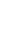# What is Volatility in Finance? - Explained Here!

Updated:26 Aug, 2022

When we discuss investments such as investment in assets like property, stocks, etc. we come around with different terms. Similarly, one of the terms which are related to investment is Volatility. So, what is Volatility? The word 'Volatility' means the quality or state of being volatile. It means the propensity to change quickly and unpredictably. Volatility is a term used to describe the fluctuation of prices within financial markets. It is a measure of how much prices change over time and is often used to measure risk. When prices are volatile, it means that they are changing rapidly and unpredictably.

Volatility is usually expressed in form of percentages. An increase in volatility means an increase in the chances of making quick money or losing as well.

## Why Should We Measure Volatility?

There are several reasons why measuring volatility is important for investors and traders.

• First, it can help you to identify opportunities. For example, if an asset or security is experiencing high levels of volatility, it may be ripe for trading.
• Second, volatility can also help you to manage risk. By understanding the level of risk associated with an asset or security, you can make more informed investment decisions and avoid potential losses.
• Finally, measuring volatility can also give you a better understanding of the overall market. By tracking the level of volatility in the market, you can get a better sense of market sentiment and make more informed investment decisions.

There are different ways to measure market volatility, but the standard deviation is mostly used and is a popular method to determine market volatility. There are some other methods also which can be used to measure market volatility such as Maximum drawdown and Beta.

The market indicators which show the volatility of the market are VIX, ATR, and Bollinger Bands. Bollinger Bands is the best market volatility indicator.

### Standard Deviation:

The most popular and common method used for measuring market volatility. The standard deviation is a measure of how much the asset's price varies from the average price. The higher the standard deviation, the greater the volatility.

It is calculated by obtaining the mean price for the specified period and then subtracting that from each price point. The differences are then Squared, Summed, and averaged to get the variance.

### Maximum Drawdown:

This metric looks at the biggest loss that investment has ever experienced, in terms of percentage.

``For example, let's say you invest in a stock that has a starting value of \$100. Over a year, the stock goes up and down in value, but it never falls below \$90. The maximum drawdown volatility for this stock would be 10%.``

Maximum drawdown volatility is important because it can give you a better understanding of the risk that you're taking on with an investment.

When you know the maximum drawdown volatility of an investment, you can compare it to other investments and see how it stacks up.

## High and Low Volatility:

If there is a rapid fluctuation in the stock price in a short period, Facing new High and Lows then it is high volatility. And, if there is a slow and steady fluctuation in the stock price, or it is most stable then it is Low volatility.

The day-to-day traders like high volatility for more opportunities to earn quickly in a short period. But long-term investors like low volatility for a steady gain.

## Volatility And Risk:

Risk is unpredictable and uncertain. It's the possibility or probability of suffering loss because of the factors that affect the overall market performance, whereas, volatility is the variation in the value of a security and the risk of high degrees of dispersion in the magnitude of securities

Overall, we can summarize that volatility is a measure of how much prices change over time and is often used to measure risk. volatility is the variation in the value of a security and the risk of high degrees of dispersion in the magnitude of securities.